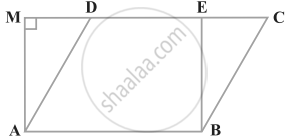# In figure if parallelogram ABCD and rectangle ABEF are of equal area, then ______. - Mathematics

MCQ
Fill in the Blanks

In figure if parallelogram ABCD and rectangle ABEF are of equal area, then ______.#### Options

• Perimeter of ABCD = Perimeter of ABEM

• Perimeter of ABCD < Perimeter of ABEM

• Perimeter of ABCD > Perimeter of ABEM

• Perimeter of ABCD = 1/2 (Perimeter of ABEM)

#### Solution

In figure if parallelogram ABCD and rectangle ABEF are of equal area, then perimeter of ABCD > Perimeter of ABEM.Explanation:

If parallelogram ABCD and rectangle ABEF are of equal area then perimeter of ABCD > Perimeter of ABEM because:

As we know that, the perpendicular distance between two parallel sides of a parallelogram is always less than the length of the other parallel sides.

BE < BC and AM < AD.

Concept: Corollary: A rectangle and a parallelogram on the same base and between the same parallels are equal in area.
Is there an error in this question or solution?

#### APPEARS IN

NCERT Mathematics Exemplar Class 9
Chapter 9 Areas of Parallelograms & Triangles
Exercise 9.1 | Q 5 | Page 86

Share